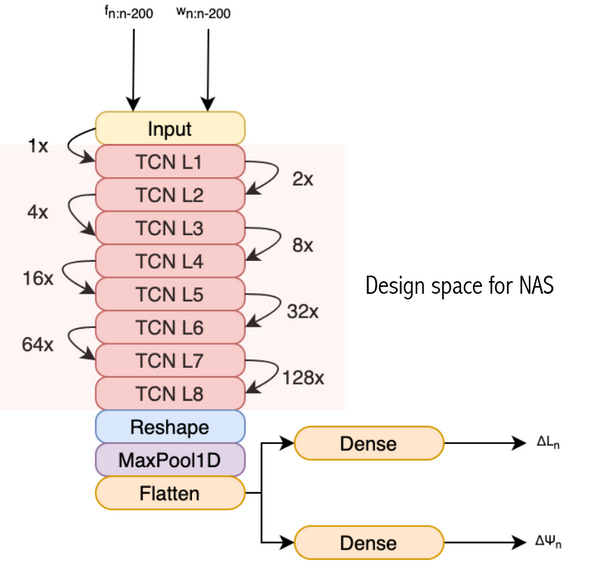# Untraced function warning and Model parsing failure for Keras TCN Regressor (TF Lite)

The error: TF Lite converter throws an untraced function warning when trying to convert a temporal CNN (built using the widely used Keras TCN library: https://github.com/philipperemy/keras-tcn ), and throws in model parsing error when trying to do post-training quantization

### 1. System information

• OS Platform and Distribution (e.g., Linux Ubuntu 16.04): Ubuntu 18.04
• TensorFlow installation (pip package or built from source): Pip (python 3.8.8)
• TensorFlow library (version, if pip package or github SHA, if built from source): 2.3.0 (TF Base), 2.4.0 (TF-GPU)

### 2. Code

Part 1, converting pretrained TF model to TF Lite Model:

``````import os
os.environ["CUDA_DEVICE_ORDER"] = "PCI_BUS_ID"
os.environ["CUDA_VISIBLE_DEVICES"]="1"
from tensorflow.python.keras.backend import set_session
import tensorflow as tf
config = tf.compat.v1.ConfigProto()
config.gpu_options.allow_growth = True  # dynamically grow the memory used on the GPU
config.log_device_placement = True  # to log device placement (on which device the operation ran)
sess = tf.compat.v1.Session(config=config)
set_session(sess)  # set this TensorFlow session as the default
import warnings
warnings.filterwarnings('ignore')

import pandas as pd
import numpy as np
from gtda.time_series import SlidingWindow
import matplotlib.pyplot as plt
from math import atan2, pi, sqrt

from tensorflow.keras.layers import Dense, MaxPooling1D, Flatten
from tensorflow.keras import Input, Model
from tensorflow.keras.callbacks import ModelCheckpoint
from tcn import TCN, tcn_full_summary
from tensorflow.keras.models import load_model

model = load_model('best_joint_new.hdf5',custom_objects={'TCN':TCN})
converter = tf.lite.TFLiteConverter.from_keras_model(model)
model_no_quant_tflite = converter.convert()
open('best_joint.tflite', "wb").write(model_no_quant_tflite)
``````

Part 2: Post training quantization

trainX is a nX200X6 matrix of floating point values, n can be any integer.

``````def representative_dataset():
for i in range(trainX.shape):
yield ([trainX[i]])
``````

Same converter used as before.

``````# Set the optimization flag.
converter.optimizations = [tf.lite.Optimize.DEFAULT]
# Enforce integer only quantization
converter.target_spec.supported_ops = [tf.lite.OpsSet.TFLITE_BUILTINS_INT8]
converter.inference_input_type = tf.int8
converter.inference_output_type = tf.int8
# Provide a representative dataset to ensure we quantize correctly.
converter.representative_dataset = representative_dataset
model_quant_tflite = converter.convert()
# Save the model to disk
open('best_joint_quant.tflite', "wb").write(model_quant_tflite)
``````

### 3. Failure after conversion

Part 1 (conversion successful but produces the following warning)

``````WARNING:absl:Found untraced functions such as residual_block_0_layer_call_and_return_conditional_losses, residual_block_0_layer_call_fn, residual_block_1_layer_call_and_return_conditional_losses, residual_block_1_layer_call_fn, residual_block_2_layer_call_and_return_conditional_losses while saving (showing 5 of 325). These functions will not be directly callable after loading.
WARNING:absl:Found untraced functions such as residual_block_0_layer_call_and_return_conditional_losses, residual_block_0_layer_call_fn, residual_block_1_layer_call_and_return_conditional_losses, residual_block_1_layer_call_fn, residual_block_2_layer_call_and_return_conditional_losses while saving (showing 5 of 325). These functions will not be directly callable after loading.
``````

Part 2 (quantization fails)

``````---------------------------------------------------------------------------
TypeError                                 Traceback (most recent call last)
~/anaconda3/envs/tflite/lib/python3.8/site-packages/tensorflow/lite/python/optimize/calibrator.py in __init__(self, model_content)
57       self._calibrator = (
---> 58           _calibration_wrapper.CalibrationWrapper(model_content))
59     except Exception as e:

TypeError: pybind11::init(): factory function returned nullptr

During handling of the above exception, another exception occurred:

ValueError                                Traceback (most recent call last)
<ipython-input-7-f34a9c965790> in <module>
7 # Provide a representative dataset to ensure we quantize correctly.
8 converter.representative_dataset = representative_dataset
----> 9 model_quant_tflite = converter.convert()
10 # Save the model to disk
11 open('best_joint_quant.tflite', "wb").write(model_quant_tflite)

~/anaconda3/envs/tflite/lib/python3.8/site-packages/tensorflow/lite/python/lite.py in convert(self)
871           graph=frozen_func.graph)
872
--> 873     return super(TFLiteKerasModelConverterV2,
874                  self).convert(graph_def, input_tensors, output_tensors)
875

~/anaconda3/envs/tflite/lib/python3.8/site-packages/tensorflow/lite/python/lite.py in convert(self, graph_def, input_tensors, output_tensors)
630     calibrate_and_quantize, flags = quant_mode.quantizer_flags()
631     if calibrate_and_quantize:
--> 632       result = self._calibrate_quantize_model(result, **flags)
633
634     flags_modify_model_io_type = quant_mode.flags_modify_model_io_type(

~/anaconda3/envs/tflite/lib/python3.8/site-packages/tensorflow/lite/python/lite.py in _calibrate_quantize_model(self, result, inference_input_type, inference_output_type, activations_type, allow_float)
447     # Add intermediate tensors to the model if needed.
448     result = _calibrator.add_intermediate_tensors(result)
--> 449     calibrate_quantize = _calibrator.Calibrator(result)
450     if self._experimental_calibrate_only or self._experimental_new_quantizer:
451       calibrated = calibrate_quantize.calibrate(

~/anaconda3/envs/tflite/lib/python3.8/site-packages/tensorflow/lite/python/optimize/calibrator.py in __init__(self, model_content)
58           _calibration_wrapper.CalibrationWrapper(model_content))
59     except Exception as e:
---> 60       raise ValueError("Failed to parse the model: %%s." %% e)
61     if not self._calibrator:
62       raise ValueError("Failed to parse the model.")

ValueError: Failed to parse the model: pybind11::init(): factory function returned nullptr.

``````

### 4. The Master Model Architecture

Input: 6 channels of 200-sample floating point values
Output: 2 scalars (floating point)### 5. Link to original HDF5 model:

https://drive.google.com/file/d/1GFRgMUkIVatSsUWgnzee_jjeF6D3l-A-/view?usp=sharingFull dicussion on github.com

## 1 possible answer(s) on “Untraced function warning and Model parsing failure for Keras TCN Regressor (TF Lite)”

1. You can ignore the above warnings if the conversion was succeeded.

Could you triage this issue, @ethkim ?